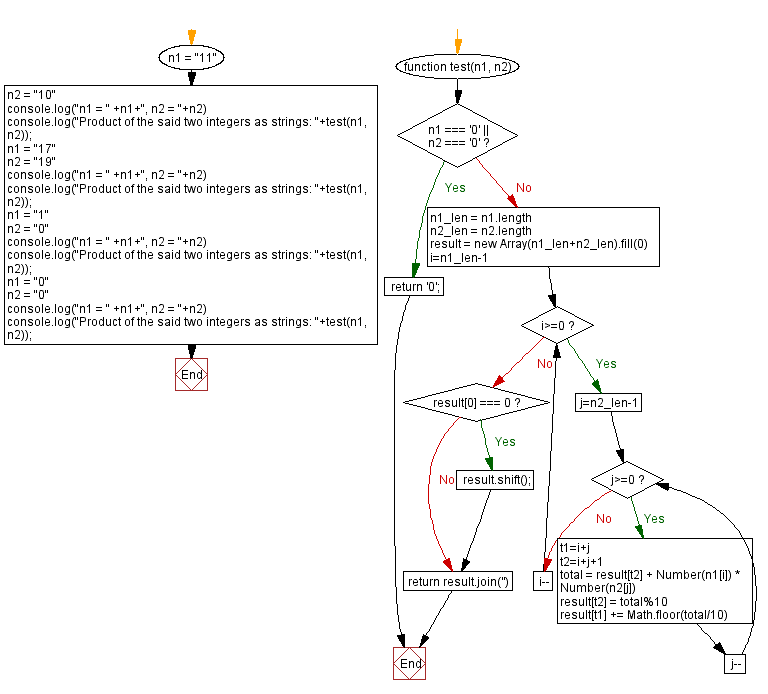# JavaScript: Product of two non-negative integers as strings

## JavaScript Math: Exercise-103 with Solution

Write a JavaScript program to calculate the product of non-negative integers n1 and n2 represented as strings. The product is also returned as a string.

Test Data:
("11", "10") -> "110"
("17", "19") -> "323"
("1", "0") -> "0"
("0", "0") -> "0"

Sample Solution:

HTML Code:

``````<!DOCTYPE html>
<html>
<meta charset="utf-8">
<title>JavaScript program to Product of two non-negative integers as strings</title>
<body>

</body>
</html>
```
```

JavaScript Code:

``````function test(n1, n2) {
if (n1 === '0' || n2 === '0')
return '0';

n1_len = n1.length
n2_len = n2.length
result = new Array(n1_len+n2_len).fill(0)

for (i=n1_len-1; i>=0; i--) {
for (j=n2_len-1; j>=0; j--) {
t1=i+j
t2=i+j+1
total = result[t2] + Number(n1[i]) * Number(n2[j])
result[t2] = total%10
result[t1] += Math.floor(total/10)
}
}
if (result === 0)
result.shift();
return result.join('')
}

n1 = "11"
n2 = "10"
console.log("n1 = " +n1+", n2 = "+n2)
console.log("Product of the said two integers as strings: "+test(n1, n2));
n1 = "17"
n2 = "19"
console.log("n1 = " +n1+", n2 = "+n2)
console.log("Product of the said two integers as strings: "+test(n1, n2));
n1 = "1"
n2 = "0"
console.log("n1 = " +n1+", n2 = "+n2)
console.log("Product of the said two integers as strings: "+test(n1, n2));
n1 = "0"
n2 = "0"
console.log("n1 = " +n1+", n2 = "+n2)
console.log("Product of the said two integers as strings: "+test(n1, n2));
```
```

Sample Output:

```n1 = 11, n2 = 10
Product of the said two integers as strings: 110
n1 = 17, n2 = 19
Product of the said two integers as strings: 323
n1 = 1, n2 = 0
Product of the said two integers as strings: 0
n1 = 0, n2 = 0
Product of the said two integers as strings: 0
```

Flowchart:Live Demo:

See the Pen javascript-math-exercise-103 by w3resource (@w3resource) on CodePen.

Improve this sample solution and post your code through Disqus

What is the difficulty level of this exercise?

Test your Programming skills with w3resource's quiz.

﻿

## JavaScript: Tips of the Day

Shorten an array using its length property

A great way of shortening an array is by redefining its length property.

```let array = [0, 1, 2, 3, 4, 5, 6, 6, 8, 9]
array.length = 4
// Result: [0, 1, 2, 3]
```

Important to know though is that this is a destructive way of changing the array. This means you lose all the other values that used to be in the array.

Ref: https://bit.ly/2LBj213